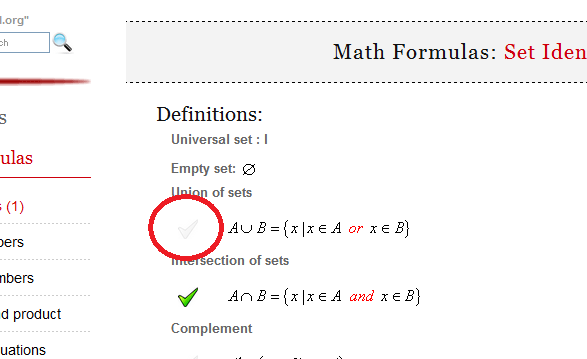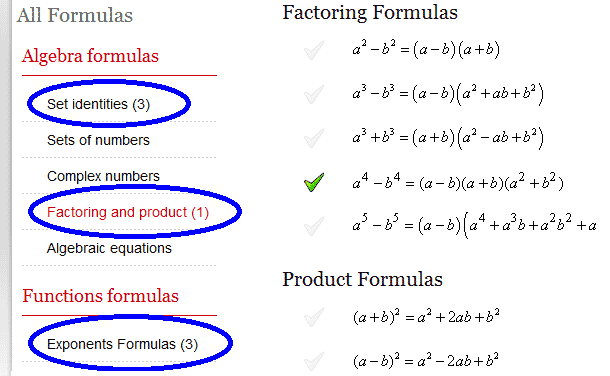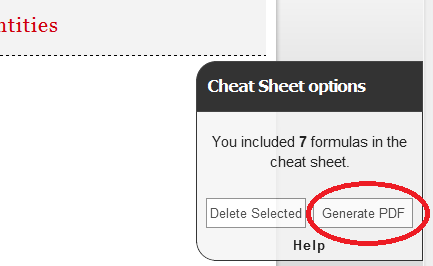Math Calculators, Lessons and Formulas

It is time to solve your math problem

mathportal.org

You included 0 formulas in your custom cheat sheet.

Cheat Sheet options

## How to generate cheat sheet ?

To create cheat sheet first you need to select formulas which you want to include in it. To select formula click at picture next to formula.You can choose formulas from different pages.After you have selected all the formulas which you would like to include in cheat sheet, click the "Generate PDF" button.# Math Formulas: Conic Sections

### The Parabola Formulas

The standard formula of a parabola

 $$y^2 = 2\,p\,x$$

Parametric equations of the parabola:

 \begin{aligned} x &=2\,p\,t^2 \\ y &= 2\,p\,t \end{aligned}

Tangent line in a point $D(x_0, y_0)$ of a parabola $y^2 = 2px$ is :

 $$y_0\,y=p\left(x+x_0\right)$$

Tangent line with a given slope $m$:

 $$y = m\,x + \frac{p}{2m}$$

Tangent lines from a given point

Take a fixed point $P(x_0, y_0)$. The equations of the tangent lines are:

 \begin{aligned} y-y_0 &= m_1(x-x_0) \\ y-y_0 &= m_2(x-x_0) \\ m_1 &= \frac{y_0 + \sqrt{y_0^2 - 2px_0}}{2x_0} \\ m_2 &= \frac{y_0 - \sqrt{y_0^2 - 2px_0}}{2x_0} \end{aligned}

### The Ellipse Formulas

The set of all points in the plane, the sum of whose distances from two fixed points, called the foci, is a constant.

The standard formula of a ellipse:

 $$\frac{x^2}{a^2} + \frac{y^2}{b^2} = 1$$

Parametric equations of the ellipse:

 \begin{aligned} x &= a\,\cos t \\ y &= b\,\sin t \end{aligned}

Tangent line in a point $D(x_0, y_0)$ of a ellipse:

 $$\frac{x_0\,x}{a^2} + \frac{y_0\,y}{b^2} = 1$$

Eccentricity of the ellipse:

 $$e = \frac{\sqrt{a^2-b^2}}{a}$$

Foci of the ellipse:

 \begin{aligned} & \text{if}~a \geq b \Longrightarrow F_1\left(-\sqrt{a^2-b^2},0\right)~~ F_2\left(\sqrt{a^2-b^2},0\right) \\ & \text{if}~a < b \Longrightarrow F_1\left(0, -\sqrt{b^2-a^2}\right) ~~ F_2\left(0, \sqrt{b^2-a^2}\right) \end{aligned}

Area of the ellipse:

 $$A = \pi \cdot a \cdot b$$

### The Hyperbola Formulas

The set of all points in the plane, the difference of whose distances from two fixed points, called the foci, remains constant.

The standard formula of a hyperbola:

 $$\frac{x^2}{a^2} - \frac{y^2}{b^2} = 1$$

Parametric equations of the Hyperbola:

 \begin{aligned} x &= \frac{a}{\sin t} \\ y &= \frac{b\,\sin t}{\cos t} \end{aligned}

Tangent line in a point $D(x_0, y_0)$ of a Hyperbola:

 $$\frac{x_0x}{a^2} - \frac{y_0y}{b^2} = 1$$

Foci:

 \begin{aligned} & \text{if}~a \geq b \Longrightarrow F_1\left(-\sqrt{a^2+b^2},0\right)~~ F_2\left(\sqrt{a^2+b^2},0\right) \\ & \text{if}~a < b \Longrightarrow F_1\left(0, -\sqrt{a^2+b^2}\right) ~~ F_2\left(0, \sqrt{a^2+b^2}\right) \end{aligned}

Asymptotes:

 \begin{aligned} & \text{if } a \geq b \Longrightarrow y = \frac{b}{a}x \text{ and } y = -\frac{b}{a}x \\ & \text{if } a < b \Longrightarrow y = \frac{a}{b}x \text{ and } y = -\frac{a}{b}x \\ \end{aligned}